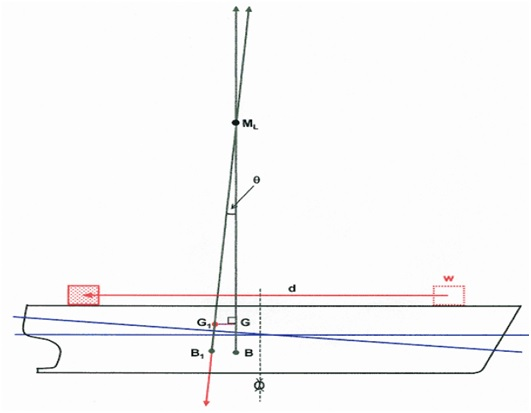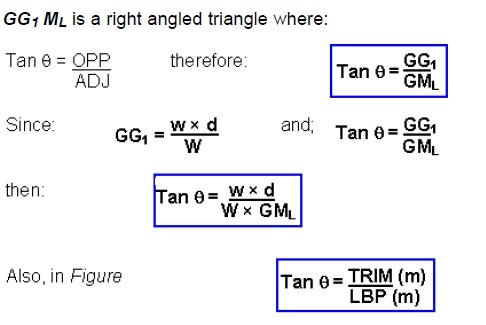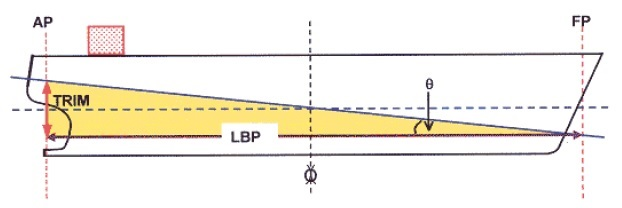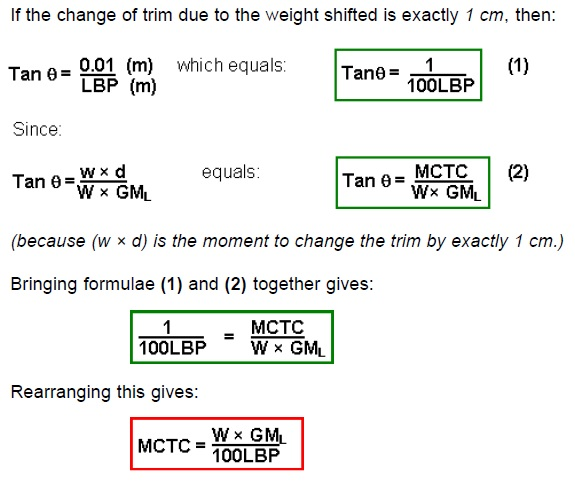# What is Trimming Moment (MCTC)?

This is the moment required to change the ships trim by exactly one centimetre.

The change of trim can be calculated by the formula: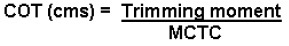where the trimming moment is: w × d
‘w’ being the weight shifted, and
‘d’ being the distance through which the weight is shifted longitudinally.

Thus: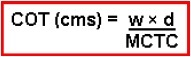FORMULA AND DERIVATION OF MCTC

In practice the MCTC value will always be found for the draught in question in the ship’s hydrostatic particulars. However, in examinations it may have to be calculated and the formula for calculating MCTC is: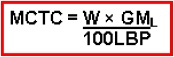where:          ‘W’ is the ship’s displacement;
‘GML’ is the longitudinal metacentric height, and;
‘LBP’ is the length between perpendiculars.

The derivation of this formula is as follows.

The ship in Figure is on an even keel with a weight on deck.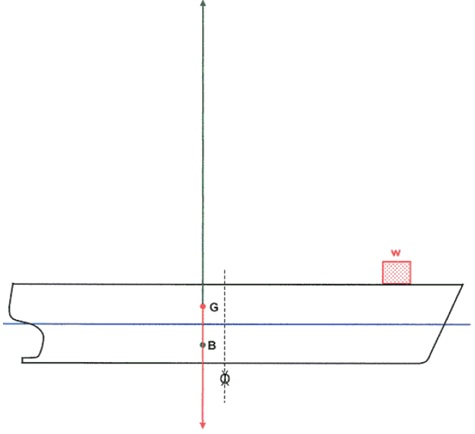The weight is shifted aft along the deck through distance ‘d’ metres. In accordance with the formula: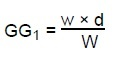G will move aft to G1 (parallel to and in the same direction as the shift of the weight).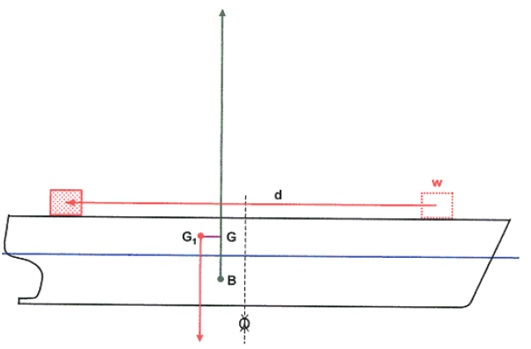G and B become horizontally separated creating a trimming lever. This causes the ship to trim by the stern until B attains a new position vertically below the new longitudinal centre of gravity, G1